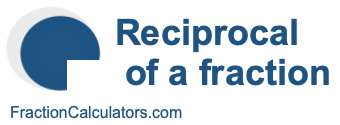Reciprocal of 2/4What is the reciprocal of 2/4? Here we will define reciprocal of 2/4, and show you how to calculate the reciprocal of 2/4 in fraction form and decimal form.

The reciprocal of 2/4 is a fraction or a number that when multiplied by 2/4 is equal to 1. To get the reciprocal of 2/4 based on that definition, we can make the following equation where "R" is the reciprocal of 2/4.

(2/4) × R = 1

When we solve for R, we get the answer to the reciprocal of 2/4 as a fraction as follows:

(2/4) × R = 1
R = 4/2
Reciprocal of 2/4 = 4/2

You can check that the answer is correct by confirming that 2/4 times the reciprocal of 2/4 is equal to 1, like this:

2/4 × 4/2 = 1

To find the decimal answer to the reciprocal of 2/4, you divide the numerator of the reciprocal by the demoninator of the reciprocal, like this:

4 ÷ 2 = 2
Reciprocal of 2/4 = 2

Tip: As you may have inferred from our tutorial above, you can quickly get the reciprocal of any fraction, such as 2/4, by switching the numerator and the denominator.

Reciprocal of a fraction
Do you need the reciprocal of another fraction? No problem! Please enter another fraction below.

Reciprocal of /

Reciprocal of 2/5
Here is the next fraction on our list that we have calculated the reciprocal of.